# The Octagonal Prism

The octagonal prism is a 3D uniform polyhedron bounded by 10 polygons (2 octagons and 8 squares), 24 edges, and 16 vertices. It may be considered to be the extrusion of the octagon.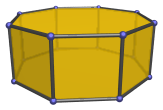The octagonal prism can be attached to a square cupola (J4) to form an elongated square cupola (J19). Attaching it to a second square cupola produces the rhombicuboctahedron, or the gyroelongated square bicupola (J37) if the second cupola is rotated 45° relative to the first.

## Projections

In order to be able to identify the octagonal prism in various projections of 4D objects, it is useful to know how it appears from various viewpoints. The following are some of the commonly-encountered views:

Projection Envelope Description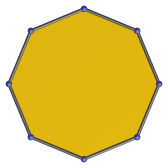Regular octagon

Octagon-centered parallel projection.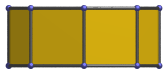Rectangle

Parallel projection centered on a vertical edge.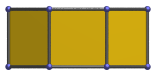Rectangle

Square-centered parallel projection.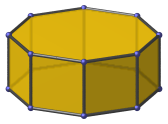Decagon

Vertex-centered parallel projection.

## Coordinates

The Cartesian coordinates of the octagonal prism, centered on the origin and having edge length 2, are:

• (±1, ±(1+√2), ±1)
• (±(1+√2), ±1, ±1)

## Occurrences

The octagonal prism occurs in the following uniform polychora:

It also occurs in a number of CRF polychora, including (but by no means limited to) the following:

Last updated 17 Jun 2019.